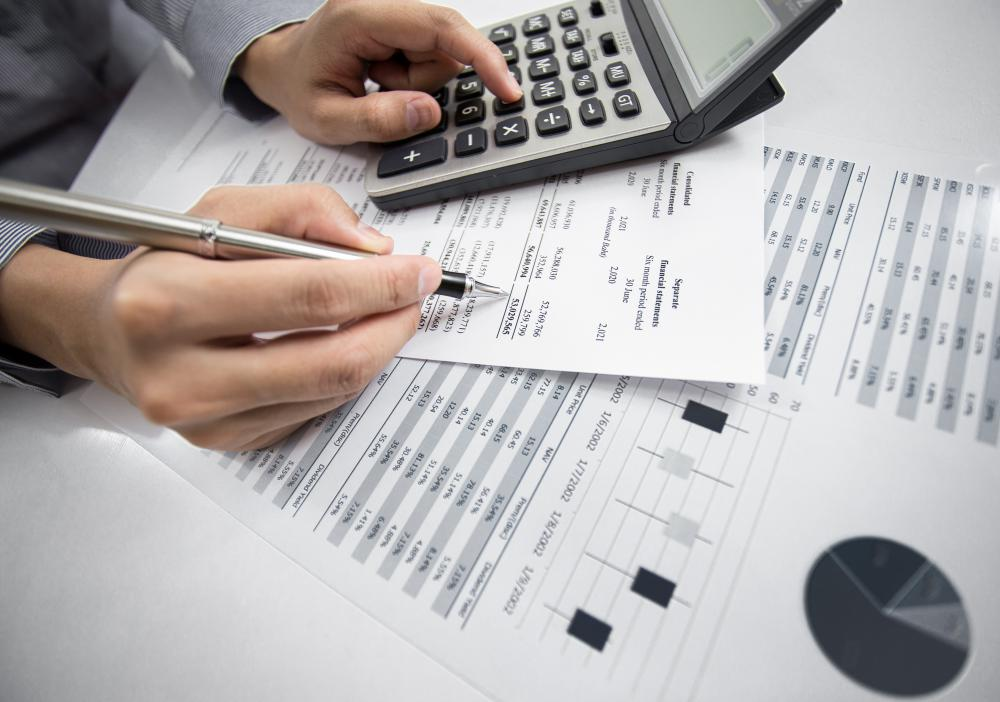# What Is the Connection between RPI and CPI?

Sandi Johnson
Sandi JohnsonWith CPI, a standard set of items purchased by the typical household, collectively known as a basket, is monitored for price fluctuations.

In economics, the consumer price index (CPI), and the retail price index (RPI) are used to measure and monitor inflation. RPI and CPI are typically calculated for a specific country or region and may carry a different common name, although the concepts are present in most modern economies. With CPI, a standard set of items purchased by the typical household, collectively known as a basket, is monitored for price fluctuations. Comparatively, RPI uses many of the same items, but might also include the cost of housing or other items not found in CPI. The primary connection between RPI and CPI, however, is their use in making economic decisions based on past and present market conditions.Typically, RPI calculations show a higher rate of inflation than CPI figures.

Different organizations use RPI and CPI differently, depending on geography, common cultural practices, government regulation, and what economic decisions need to be made. Some organizations, such as government bodies, use CPI to determine economic policies and set government benefit amounts. Other organizations, such as businesses, use RPI to make investment recommendations or determine pension benefits for retirees. Likewise, some organizations use both figures, opting for one over the other, depending on the economic decision at hand. For example, the government in the United Kingdom bases benefit payments on CPI figures, but bases taxation and tax rates on RPI figures.

Although both RPI and CPI are used to measure inflation in a given economic area, the methods for calculating the rate of increase or decrease is different. Formulas used to calculate RPI are typically simple arithmetic formulas based on average. All of the items used in the basket are added, then divided by the total number of items. Alternatively, calculating CPI involves a more complex approach, known as the geometric mean. This approach uses multiplication and root values to reach an average result, expressed in percentages.

Typically, RPI calculations show a higher rate of inflation than CPI figures. According to economic experts, the average difference between RPI and CPI is approximately one percent. If CPI was calculated using the same formula as RPI, for example, then CPI results would typically be around one percent higher than results using geometric mean formulas. Similarly, if RPI was calculated using geometric mean, the results would typically indicate a lower rate of inflation than results calculated using arithmetic mean.

Arguments regarding the difference in accuracy rates between RPI and CPI are common among economic experts. Many argue accuracy based on what items are used in RPI and CPI. Still others argue accuracy based on the formulas used to calculate each figure. Few countries regulate which figure businesses must use, although many governments officially declare which method is used in making public policy decisions.

## You might also Like